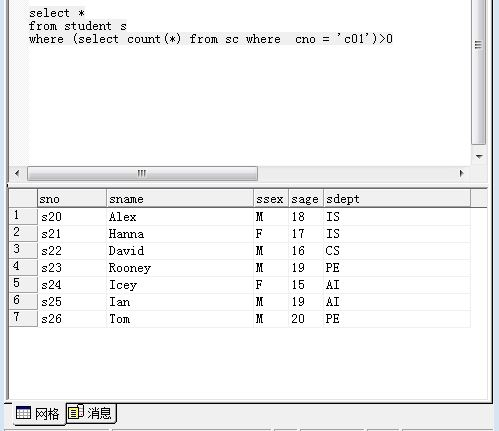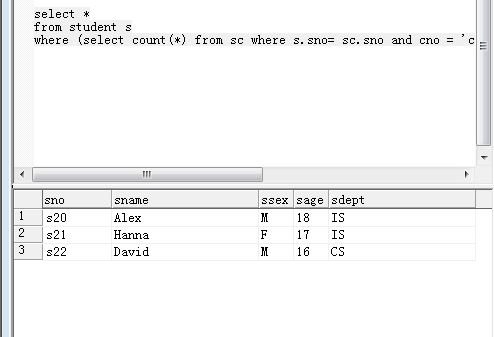``````select *
from student s
where (select count(*) from sc where  cno = 'c01')>0
``````select *
from student s
where (select count(*) from sc where s.sno= sc.sno and cno = 'c01')>0select*
from student，sc
where student.sno = sc.sno

6个回答

select * from student s where (select count(*) from sc where s.sno= sc.sno and cno = 'c01')>0；2 年多之前 回复2 年多之前 回复gzrjzcx 谢谢您的回答，不过感觉错误不一样吧？
2 年多之前 回复gzrjzcx 当然，我就是不明白第二个加入表连接的条件后为什么会有不一样的结果？
2 年多之前 回复2 年多之前 回复2 年多之前 回复

select * from students where (select count(* ) from sc where cno = 'c01' )>0

sc表有数据，所有(select count(* ) from sc where cno = 'c01' )>0 恒成立gzrjzcx 谢谢回答，我也是这么想的。但是select * from students where (select count(* ) from sc where student.sno = sc.sno and cno = 'c01' )>0 也就是加上连接表的条件过后，为什么就可以得到不一样的效果？
2 年多之前 回复